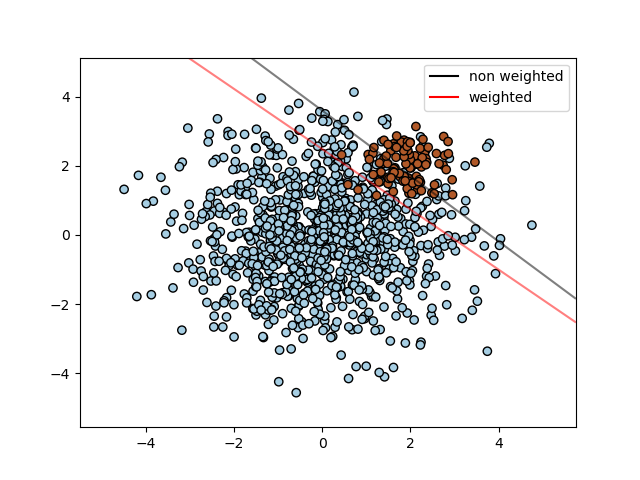# SVM: Separating hyperplane for unbalanced classes¶

Find the optimal separating hyperplane using an SVC for classes that are unbalanced.

We first find the separating plane with a plain SVC and then plot (dashed) the separating hyperplane with automatically correction for unbalanced classes.

Note

This example will also work by replacing SVC(kernel="linear") with SGDClassifier(loss="hinge"). Setting the loss parameter of the SGDClassifier equal to hinge will yield behaviour such as that of a SVC with a linear kernel.

For example try instead of the SVC:

clf = SGDClassifier(n_iter=100, alpha=0.01)import matplotlib.pyplot as plt
from sklearn import svm
from sklearn.datasets import make_blobs
from sklearn.inspection import DecisionBoundaryDisplay

# we create two clusters of random points
n_samples_1 = 1000
n_samples_2 = 100
centers = [[0.0, 0.0], [2.0, 2.0]]
clusters_std = [1.5, 0.5]
X, y = make_blobs(
n_samples=[n_samples_1, n_samples_2],
centers=centers,
cluster_std=clusters_std,
random_state=0,
shuffle=False,
)

# fit the model and get the separating hyperplane
clf = svm.SVC(kernel="linear", C=1.0)
clf.fit(X, y)

# fit the model and get the separating hyperplane using weighted classes
wclf = svm.SVC(kernel="linear", class_weight={1: 10})
wclf.fit(X, y)

# plot the samples
plt.scatter(X[:, 0], X[:, 1], c=y, cmap=plt.cm.Paired, edgecolors="k")

# plot the decision functions for both classifiers
ax = plt.gca()
disp = DecisionBoundaryDisplay.from_estimator(
clf,
X,
plot_method="contour",
colors="k",
levels=,
alpha=0.5,
linestyles=["-"],
ax=ax,
)

# plot decision boundary and margins for weighted classes
wdisp = DecisionBoundaryDisplay.from_estimator(
wclf,
X,
plot_method="contour",
colors="r",
levels=,
alpha=0.5,
linestyles=["-"],
ax=ax,
)

plt.legend(
[disp.surface_.collections, wdisp.surface_.collections],
["non weighted", "weighted"],
loc="upper right",
)
plt.show()


Total running time of the script: ( 0 minutes 0.178 seconds)

Gallery generated by Sphinx-Gallery